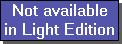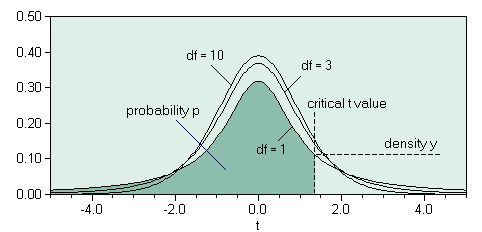The SDL Component Suite is an industry leading collection of components supporting scientific and engineering computing. Please visit the SDL Web site for more information....## tDistriIntegralUnit: SDL_statis Class: none Declaration: function tDistriIntegral (t: double; df : integer): double;

The function tDistriIntegral returns the integral of the t-distribution between minus infinity and the value of the parameter t. The parameter df specifies the number of degrees of freedom. The returned value may vary between 0.0 and 1.0.Hint 1: The calculated value is exact to about 8 decimal places.

 Hint 2: The function tDistriIntegral calculates the integral from 0.0 to the t value. This differs from the convention of how the integral of the t distribution is used in many statistical packages, which tabulate the integral between +infinity and the t value. Thus you have to use 1-tDistriIntegral to get comparable results. For example, in order to calculate the function TDIST(x,df) of Microsofts Excel® you have to use 1-tDistriIntegral(x,df).

 Hint 3: Background information on the t-distribution can be found in Fundamentals of Statistics

Last Update: 2020-Aug-23